# Row vs. Column; What is the Difference Between a Row and a Column in a Spreadsheet?

TL;DR

Rows in spreadsheets refer to the range of horizontal cells from left to right on a spreadsheet and columns in spreadsheets refer to the range vertical cells from top to bottom. Rows and columns are combined to identify cells and ranges of cells.

Every major spreadsheet software (Google Sheets, Microsoft Excel, Numbers etc.) all utilize a rows and columns system.  Rows and columns are the foundational building blocks of all spreadsheets and it's critical that you understand the difference when making your best spreadsheet.

Columns in spreadsheets refer to the range of vertical cells from top to bottom

Columns are identified by letters starting with A and move in alphabetical order as you move to the right. A is the leftmost column, B is the column immediately to its right, C is to the right of column B, etc.

The maximum number of columns possible in a worksheet is 16,384.

A straight vertical column starting with the first cells in a spreadsheet would be A:A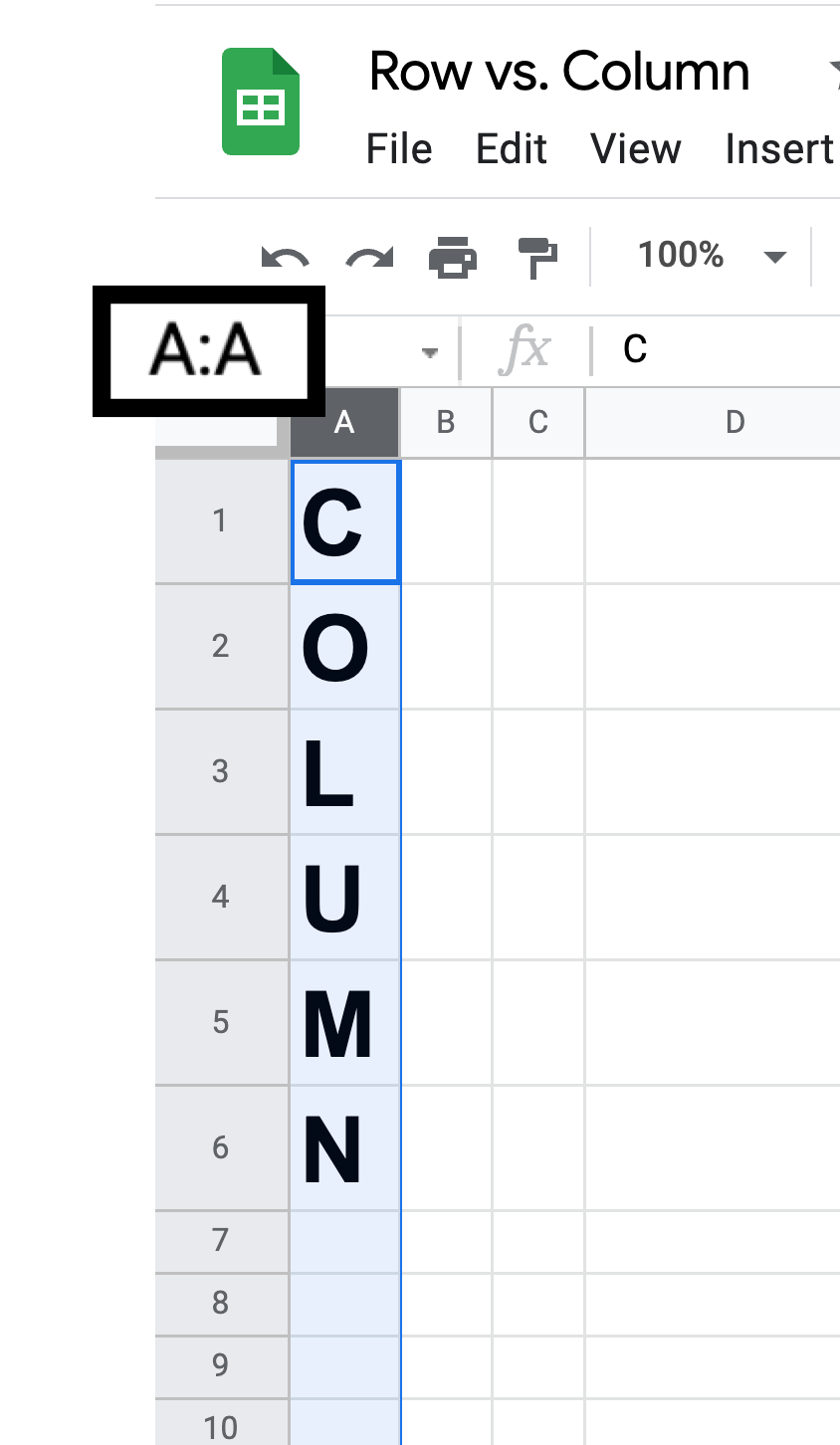If you have more columns than 26 (A-Z), columns become labeled as AA, AB, AC etc.

Once you go past the next 26, the prefix of the column moves to the next letter in the alphabet, B and the pattern continues. BA, BB, BC etc.

The size of a column is measured in width.

Rows in spreadsheets refer to the range of horizontal cells from left to right on a spreadsheet.

Rows are identified by numbers starting with 1 and can go up to 1,048,576 total.

The first row of a spreadsheet would be identified as 1:1.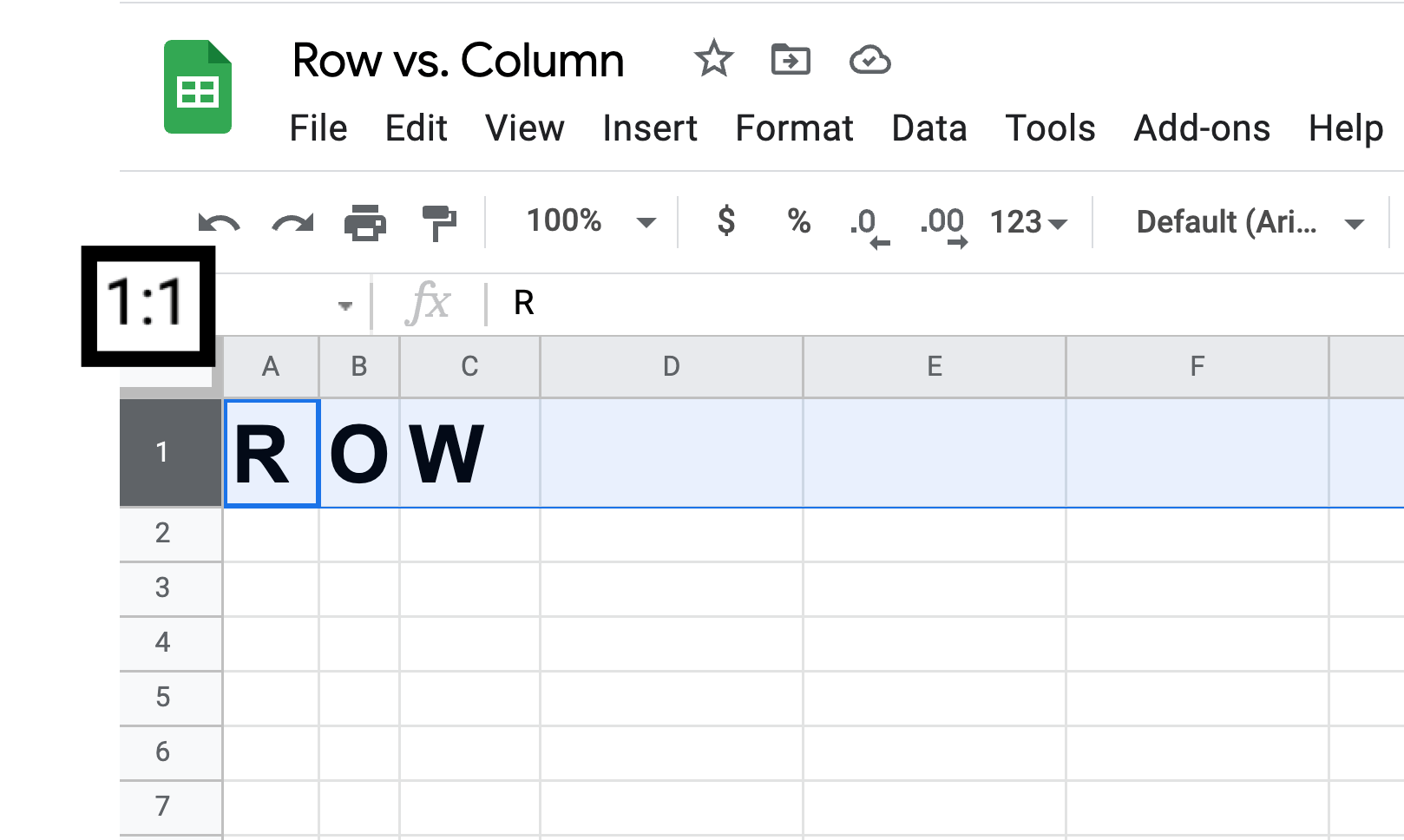The size of a row is measured in height.

## Columns + Rows = Cells

Rows and columns work like coordinates to help us identify cells.

The order of a cell is column + row.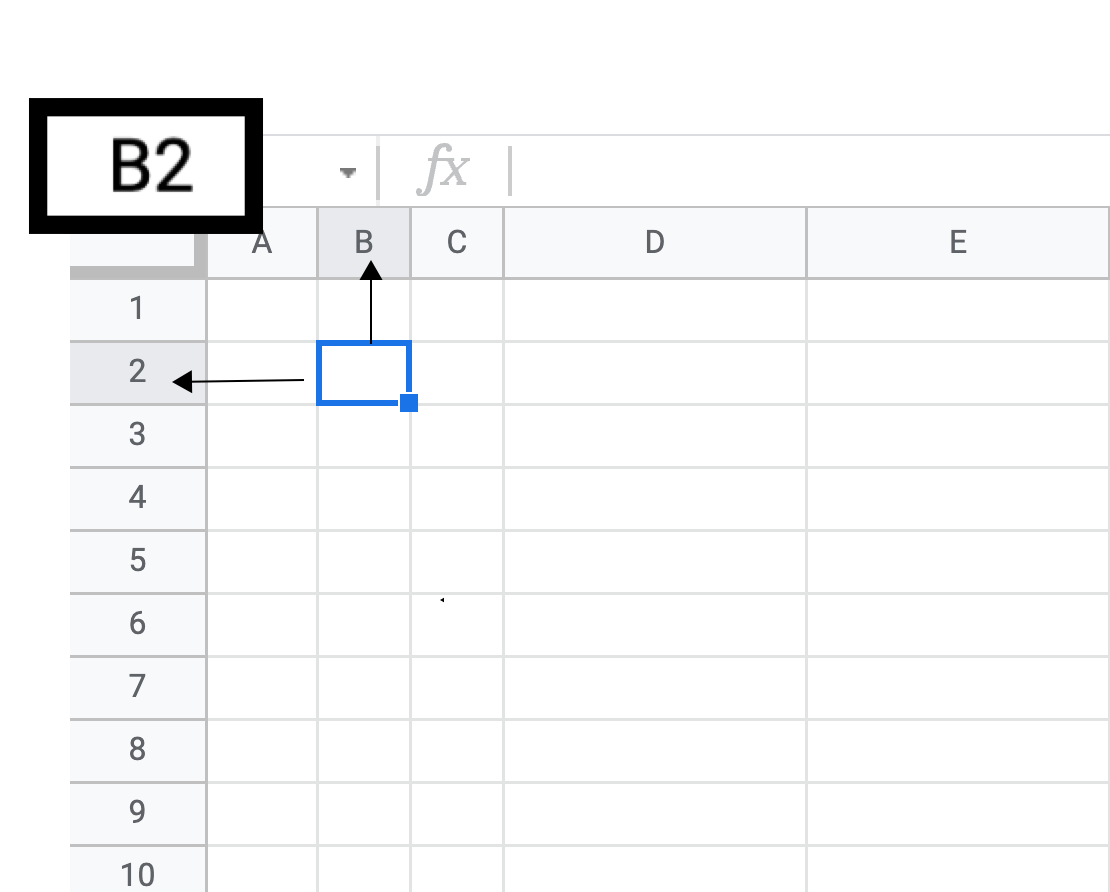Cell B2 in the example below is the location of the cell at the intersection of column B (vertical) and row 2 (horizontal).

## Ranges vs. Columns or Rows

Rows refer to the leftmost cell all the way to the rightmost cell. If we want to identify a certain group of cells that are horizontal but do not go all the way across, this is called a range.

A range is identified similar to a cell but it starts with the upper/leftmost cell of the area and ends a the bottom/rightmost cell of the area. The example below is a vertical range but isn't a row because it doesn't go all the way across. It is labeled as A1:F1.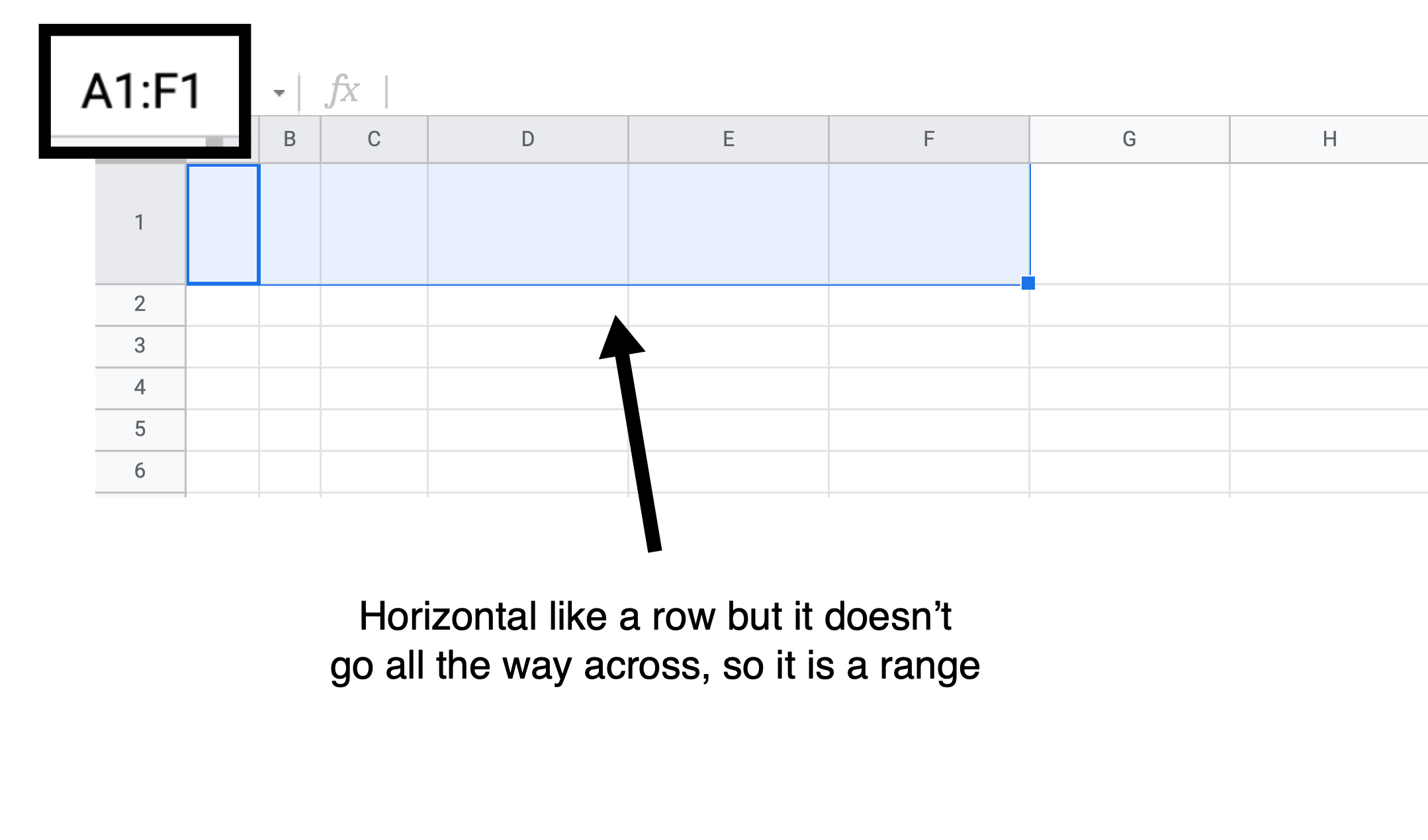Likewise, columns refer to the topmost cell all the way to the bottommost cell. Anything that falls in between this is called a range.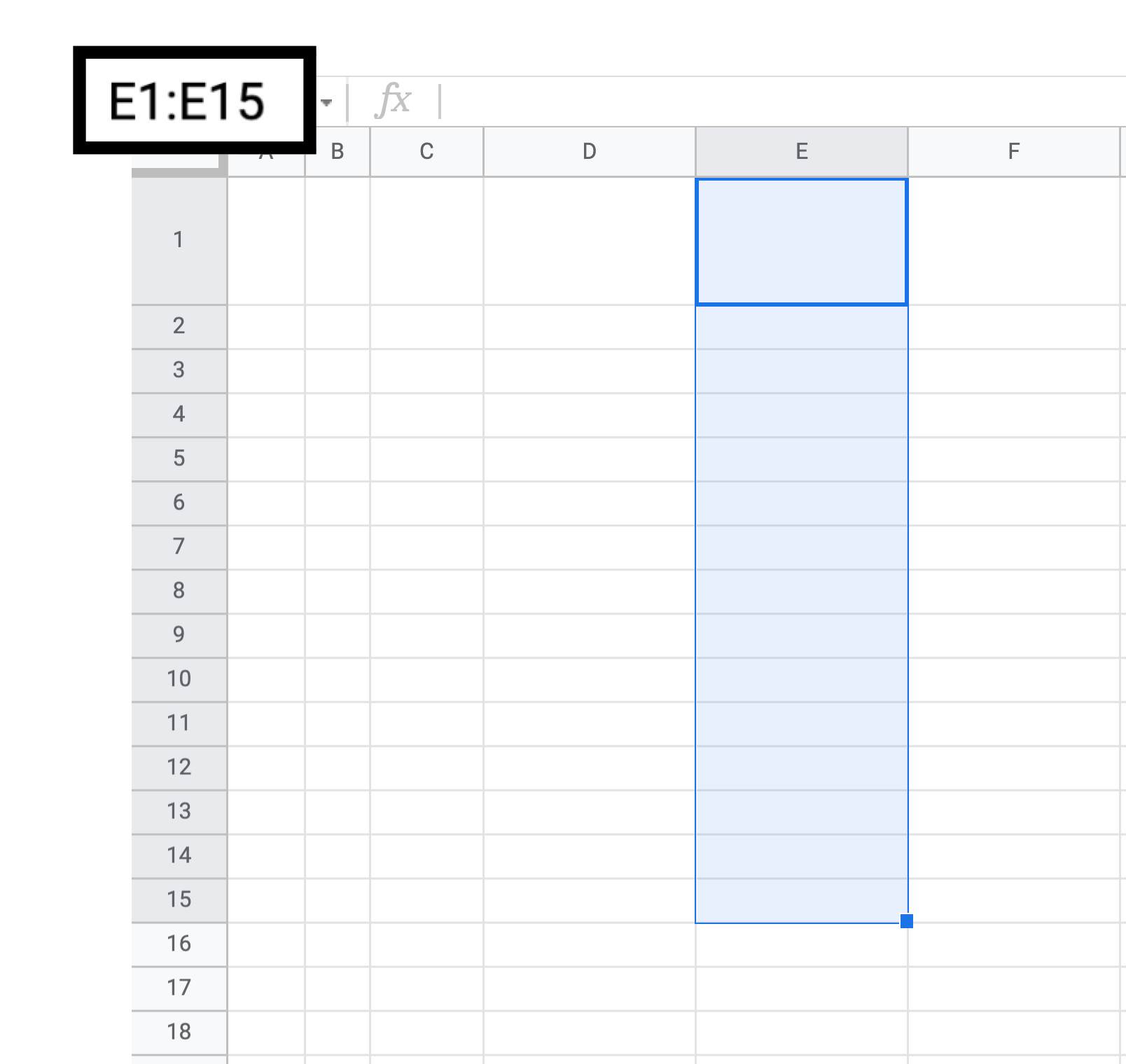## Column vs. Row Comparison

Column

Row

Vertical

Horizontal

Identified with letters

Identified with numbers

Measured in width

Measured in height

Max number = 16,384

Max number = 1,048,576

Do you have questions about rows vs. columns? Let us know in the comments!